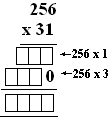Name: ___________________Date:___________________

 Email us to get an instant 20% discount on highly effective K-12 Math & English kwizNET Programs!

### Grade 5 - Mathematics1.36 Multiplication of Two or More Digits - Illustrated

 Method: Read the following questions carefully. Multiply and write the result. If the factors contain zeroes, add the same number of zeroes to the product obtained by multiplying the non-zero numbers. Example:Multiply the non zero numbers 6 and 7 which gives 42. Count the number of zeroes in the factors, there are two. Two zeroes are added to the product. Answer: 4200 Example:Multiply the as shown in the picture. The 3 digits on top is multiplied with every digit in the second number. Multiply 256 by 3 in ones place, 2 in tens place and 1 in hundreds place. Add the products. Answer: 31488 Directions: Multiply the following. Also write atleast 5 examples of your own.Name: ___________________Date:___________________

### Grade 5 - Mathematics1.36 Multiplication of Two or More Digits - Illustrated

 Q 1: Find the answer.3148831994188 Q 2: Find the answer.687673977936 Q 3: Find the answer.430045004200 Q 4: Find the answer.315031500315000 Question 5: This question is available to subscribers only! Question 6: This question is available to subscribers only!